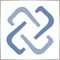89

I'm trying to add volume in a function like this:

double vol(int o,int k,const long &tick_volume2[])
{

for(int i=0;i<o;i++)
{
Buffer3[k] += (double) tick_volume2[k-o];

}
return(Buffer3[k]);
}

This works but the problem is that "+=" means that the active candle goes crazy. With every tick, the volume adds itself so the graph goes crazy rather than count all the ticks at the end of the candle.

Does anybody have any idea how to stabilize the live section of the graph?1419

Hint, you can write your function easier:)

return(Buffer3[k] += (double) tick_volume2[k-o] * o);